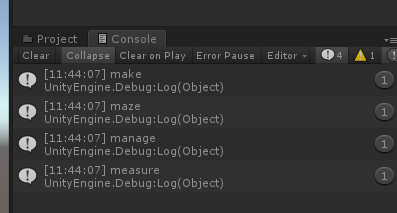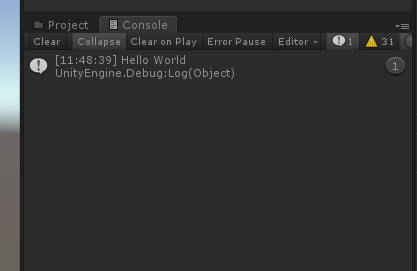# 【Unity3D日常开发】在Unity中使用正则表达式

## 二、Unity使用正则表达式

using System.Text.RegularExpressions;
using UnityEngine;

public class Regex_Test : MonoBehaviour
{
void Start()
{
string temp = "123";
Debug.Log(IsNumber(temp));
}

///<summary>
///匹配正整数
///</summary>
///<param name="strInput"></param>
///<returns></returns>
public bool IsNumber(string strInput)
{
Regex reg = new Regex("^[0-9]*[1-9][0-9]*$"); if (reg.IsMatch(strInput)) { return true; } else { return false; } } }  结果：匹配大写字母 using System.Text.RegularExpressions; using UnityEngine; public class Regex_Test : MonoBehaviour { void Start() { string temp = "ABC"; Debug.Log(IsNumber(temp)); } ///<summary> ///匹配由26个英文字母的大写组成的字符串 ///</summary> ///<param name="strInput"></param> ///<returns></returns> public bool IsCapital(string strInput) { Regex reg = new Regex("^[A-Z]+$");
if (reg.IsMatch(strInput))
{
return true;
}
else
{
return false;
}
}
}## 三、Regex 类

Regex 类用于表示一个正则表达式。

1public bool IsMatch( string input )指示 Regex 构造函数中指定的正则表达式是否在指定的输入字符串中找到匹配项。
2public bool IsMatch( string input, int startat )指示 Regex 构造函数中指定的正则表达式是否在指定的输入字符串中找到匹配项，从字符串中指定的开始位置开始。
3public static bool IsMatch( string input, string pattern )指示指定的正则表达式是否在指定的输入字符串中找到匹配项。
4public MatchCollection Matches( string input )在指定的输入字符串中搜索正则表达式的所有匹配项。
5public string Replace( string input, string replacement )在指定的输入字符串中，把所有匹配正则表达式模式的所有匹配的字符串替换为指定的替换字符串。
6public string[] Split( string input )把输入字符串分割为子字符串数组，根据在 Regex 构造函数中指定的正则表达式模式定义的位置进行分割。

## 四、常用正则表达式

^-?\d+$//匹配整数（包含正负整数） ^(-?\d+)(\.\d+)?$　 //匹配浮点数（包含正负浮点数）
^[A-Za-z]+$//匹配26个英文字母（包含大小写） ^[A-Z]+$　　		//匹配由26个英文字母（大写）
^[a-z]+$//匹配由26个英文字母（小写） ^[A-Za-z0-9]+$　　	//匹配由数字和26个英文字母组成的字符串
^\w+$//匹配由数字、26个英文字母或者下划线组成的字符串 \S{6,} //不能为空 六位以上 [^\x00-\xff] //匹配双字节字符(包括汉字在内) \d+\.\d+\.\d+\.\d+ //匹配ip地址  序号代码描述 1^\d+$匹配非负整数（正整数 + 0）
2^[0-9]*[1-9][0-9]*$匹配正整数 3^((-\d+)|(0+))$匹配非正整数（负整数 + 0）
4^-[0-9]*[1-9][0-9]*$匹配负整数 5^-?\d+$匹配整数
6^\d+(\.\d+)?$匹配非负浮点数（正浮点数 + 0） 7^(([0-9]+\.[0-9][1-9][0-9])|([0-9][1-9][0-9].[0-9]+)|([0-9][1-9][0-9]))$匹配正浮点数
8^((-\d+(.\d+)?)|(0+(.0+)?))$匹配非正浮点数（负浮点数 + 0） 9^(-(([0-9]+.[0-9][1-9][0-9])|([0-9][1-9][0-9].[0-9]+)|([0-9][1-9][0-9])))$匹配负浮点数
10^(-?\d+)(.\d+)?$匹配浮点数 11^[A-Za-z]+$匹配由26个英文字母组成的字符串
12^[A-Z]+$匹配由26个英文字母的大写组成的字符串 13^[a-z]+$匹配由26个英文字母的小写组成的字符串
14^[A-Za-z0-9]+$匹配由数字和26个英文字母组成的字符串 15^\w+$匹配由数字、26个英文字母或者下划线组成的字符串
16^[\w-]+(.[\w-]+)*@[\w-]+(.[\w-]+)+$匹配email地址 17^[a-zA-z]+://匹配(\w+(-\w+))(.(\w+(-\w+)))(?\S)?$匹配url
18[\u4e00-\u9fa5]匹配中文字符的正则表达式
19[^\x00-\xff]匹配双字节字符(包括汉字在内)
20String.prototype.len=function(){return this.replace([^\x00-\xff]/g,“aa”).length;}应用：计算字符串的长度（一个双字节字符长度计2，ASCII字符计1）
21\n[\s| ]*\r匹配空行的正则表达式
22/<(.*)>.</\1>|<(.) />匹配HTML标记的正则表达式
23(^\s*)|(\s*\$)匹配首尾空格的正则表达式

## 五、实例

### 实例 1 、匹配以 ‘m’ 开头以 ‘e’ 结尾的单词

using System.Text.RegularExpressions;
using UnityEngine;

public class Regex_Test : MonoBehaviour
{
void Start()
{
string temp = "make maze and manage to measure it";
MatchStr(temp);
}

public void MatchStr(string str)
{
Regex reg = new Regex(@"\bm\S*e\b");
MatchCollection mat = reg.Matches(str);
foreach (Match item in mat)
{
Debug.Log(item);
}
}
}### 实例2、 替换掉多余的空格

using System.Text.RegularExpressions;
using UnityEngine;

public class Regex_Test : MonoBehaviour
{
void Start()
{
string temp = "Hello              World";
MatchStr(temp);
}

public void MatchStr(string str)
{
Regex reg = new Regex("\\s+");
Debug.Log(reg.Replace(str, " "));
}
}09-07134
09-18158301-1078
11-201130
12-031345
04-0180
10-278443
03-049069
11-121494
01-25220点击重新获取扫码支付余额充值